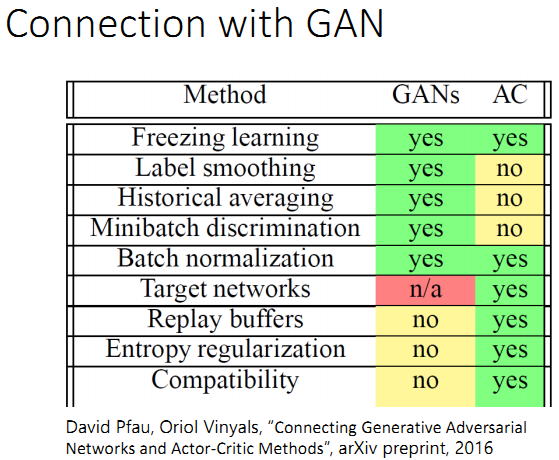2019-03-18 09:23:24 cindy_1102 阅读数 3479

# Q-Learning介绍

## 基本思想

Q-learning – value-base

critic并不直接决定采取什么行动，但是会用来衡量一个actor的好坏
critic的输出值取决于被评估的actor

1. 基于蒙特卡洛的方法Monte-Carlo (MC)
critic 观察 $\pi$ 进行游戏的整个过程, 直到该游戏回合结束再计算累积收益（通过比较期望收益和实际收益G，来训练critic）
Tip: 有时一个游戏回合可能会很长，这个等到游戏回合结束再计算收益的方法训练起来会很慢，因此引入另外一种方法 Temporal-difference（TD）
2. 时序分差方法Temporal-difference (TD)
时序分差算法计算的是两个状态之间的收益差. （通过比较期望差异与实际差异r之间的差别来训练critic）

MC vs. TD

## Q-Learning:

1. 使用一个初始的actor $\pi$ 与环境进行互动
2. 学习该actor对应的 Q function
3. 一定存在另外一个表现更好的actor $\pi'$ , 用这个更好的actor来替代原来的actor
4. 重复上述步骤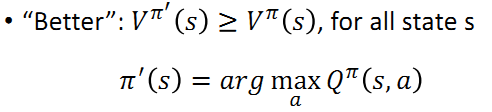Tips:

1. $\pi'$ 不包含额外的参数，它只取决于Q
2. 对于连续的action不适用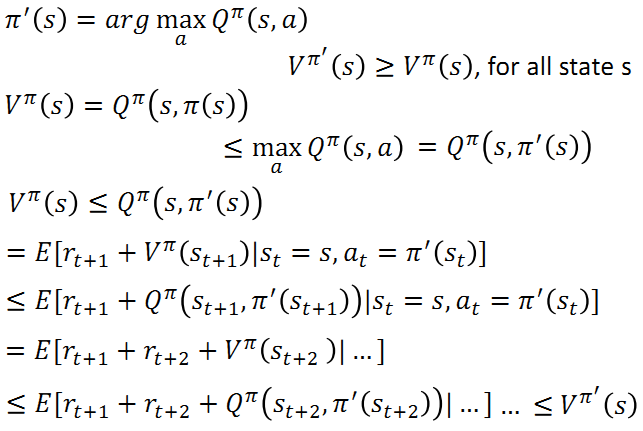Tip1: Target network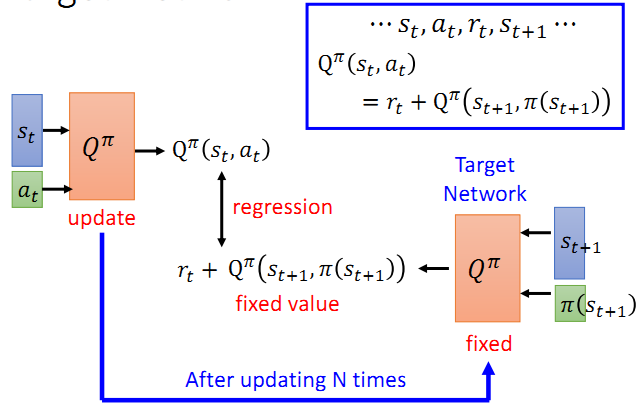Tip2: Exploration

1. Epsilon Greedy (在训练的过程中 $\epsilon$ 的值会逐渐减小)
下述公式的含义是，在采取action的时候，actor会有 $1-\epsilon$ 的概率选择使得Q值最大的a，随着训练时间变长，$\epsilon$ 的值逐渐减小，在后期actor选择最大Q值对应的a才会变大。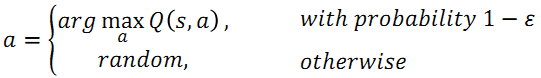2. Boltzmann Exploration (和 policy gradient 类似, 根据一个概率分布来进行采样)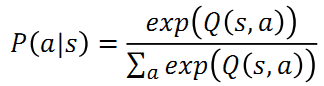Tip3: Replay Buffer
step1:$\pi$ 和环境互动
step2: 将步骤1中互动得到的经验放入一个 buffer (放在buffer里面的经验来自不同的policy，当buffer满了的时候，移除旧的经验)
(这里所说的经验是指集合 ${s_t, a_t, r_t, s_{t+1}}$)
step3: 在每一次迭代中，学习 $Q^\pi (s,a)$: 1. 部分采样 2. 更新 Q-function
step4: 找到一个比 $\pi$ 更好的 $\pi'$
step5: 重复上述步骤

1. 给定状态state $s_t$，基于使用epsilon greedy的Q采取行动action $a_t$
2. 得到对应的回报reward $r_t$ 以及新的状态state $s_{t+1}$
3. 将收集到的$\{s_t, a_t, r_t, s_{t+1}\}$存入reply buffer
4. 从reply buffer当中任意采样得到$\{s_i, a_i, r_i, s_{i+1}\}$（通常是取一部分样本）
5. 目标值为 $y = r_i + max_{a} \hat{Q}(s_{i+1},a)$
6. 根据目标值进行回归，不断更新Q的参数，使得计算出来的 $Q(s_i, a_i)$ 接近于真实值y
7. 每C步更新一次 $\hat{Q} = Q$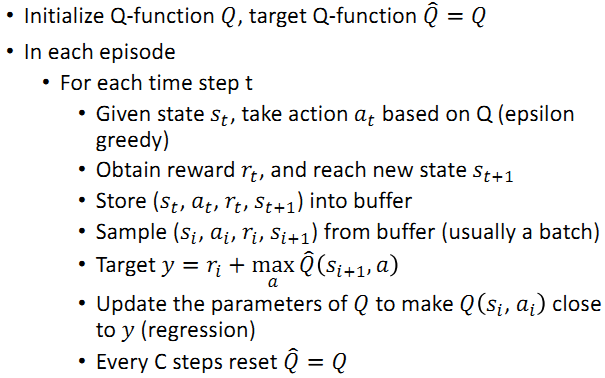## 关于Q-Learning 的几点建议

Double DQN

double DQN的真实Q值往往比Q-learning高

1. 为什么 Q 经常被高估
因为目标值 $r_t+maxQ(s_{t+1}, a)$ 总是倾向于选择被高估的行动action
2. double DQN 是如何工作的?
使用两个Q function（因此称为double）, 一个用来选择行动action，另外一个用来计算Q值，通常会选择target network来作为另外一个用于计算Q值的Q‘ function.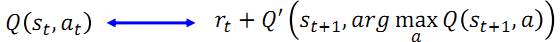如果Q高估了 a 从而被选择， Q’ 会给这个被选择的a一个合适的Q值
入股Q’会高估某个action a，这个action并不会被Q选择到

Dueling DQN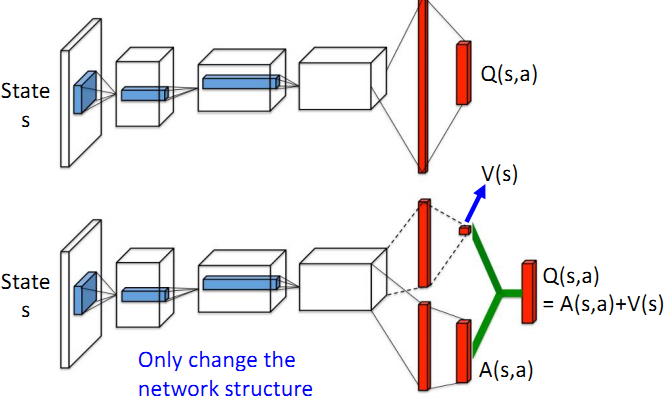V(s): 表示静态环境，状态s所具有的价值.

Prioritized Experience Replay

Multi-step: Combination of MC and TD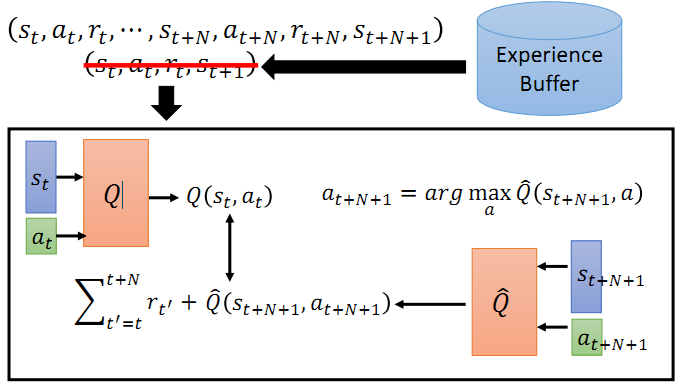Noisy Net:
Epsilon Greedy vs. Noisy Net
Epsilon Greedy: 在行动上加噪声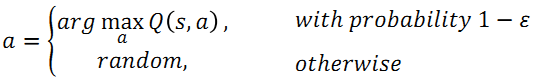Noisy Net: 在参数上加噪声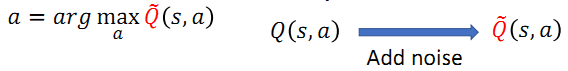Distributional Q-function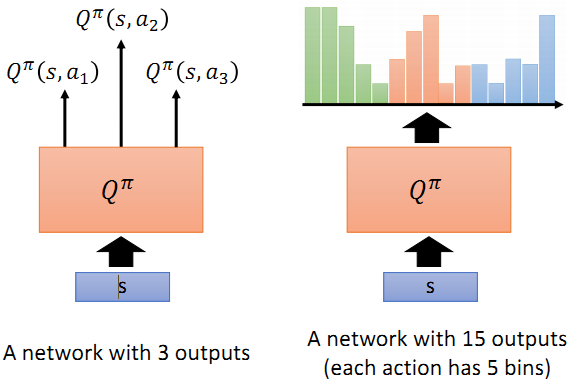Distributional Q-function 认为可以输出Q值的分布，当具有相同的均值时，选择具有较小方差（风险）的那一个

Rainbow: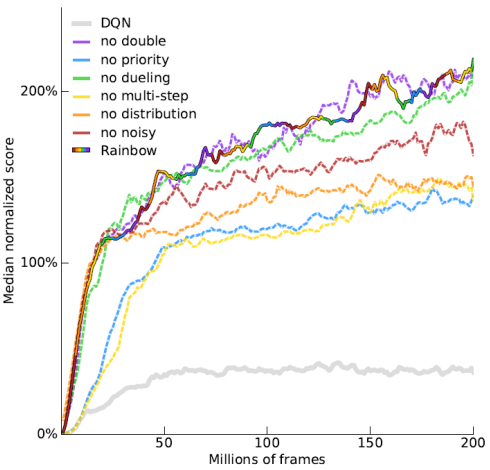## 连续行动下的 Q-Learning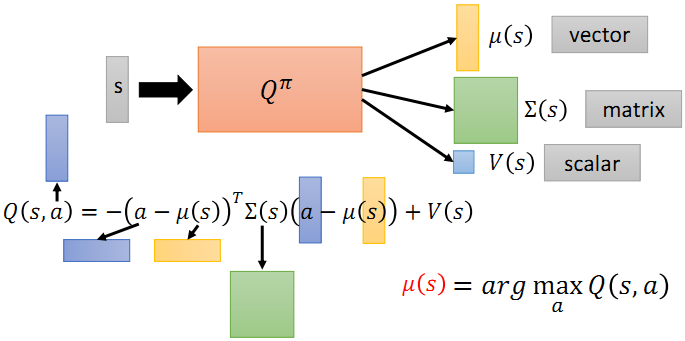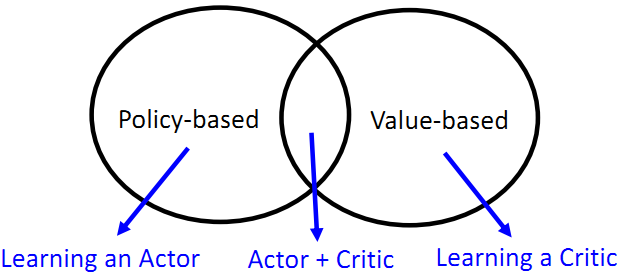2019-07-13 11:06:32 csdn_47 阅读数 98

## 术语和基本思想

• 环境 environment (给定的，无法控制)
• 回报函数 reward function (无法控制)

Policy of actor π: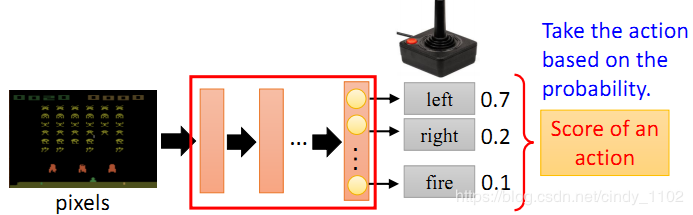Episode:

actor的目标:

Trajectory $\tau$:

$\begin{array} { l } { p _ { \theta } ( \tau ) } \\ { \quad = p \left( s _ { 1 } \right) p _ { \theta } \left( a _ { 1 } | s _ { 1 } \right) p \left( s _ { 2 } | s _ { 1 } , a _ { 1 } \right) p _ { \theta } \left( a _ { 2 } | s _ { 2 } \right) p \left( s _ { 3 } | s _ { 2 } , a _ { 2 } \right) } \\ { \quad = p \left( s _ { 1 } \right) \prod _ { t = 1 } ^ { T } p _ { \theta } \left( a _ { t } | s _ { t } \right) p \left( s _ { t + 1 } | s _ { t } , a _ { t } \right) } \end{array}$

$\overline { R } _ { \theta } = \sum _ { \tau } R ( \tau ) p _ { \theta } ( \tau ) = E _ { \tau \sim p _ { \theta } ( \tau ) } [ R ( \tau ) ]$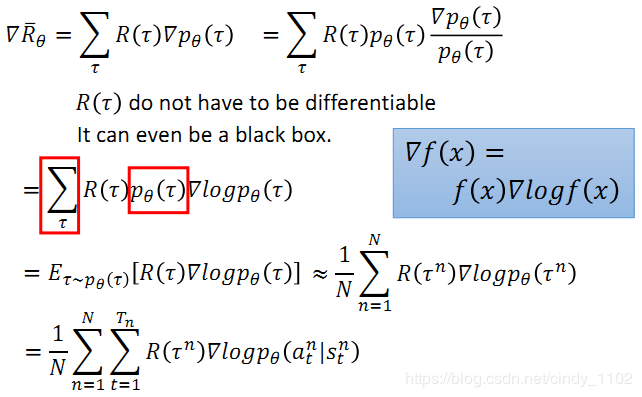### Tip 1: 添加基准线

$\nabla \overline { R } _ { \theta } \approx \frac { 1 } { N } \sum _ { n = 1 } ^ { N } \sum _ { t = 1 } ^ { T _ { n } } \left( R \left( \tau ^ { n } \right) = b \right) \nabla \log p _ { \theta } \left( a _ { t } ^ { n } | s _ { t } ^ { n } \right) \quad b \approx E [ R ( \tau ) ]$

### Tip 2: 进一步考虑各个时间点的累积收益计算方式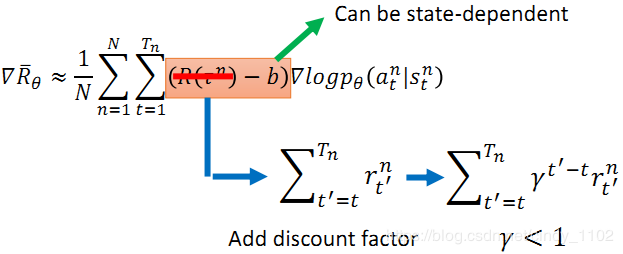2019-03-01 11:15:41 cindy_1102 阅读数 1097

# 为什么要模仿学习 Imitation Learning

(也称为 learning by demonstration, apprenticeship learning)

1. 机器也可以和环境进行互动，但是不能明显的得到reward
2. 在某些任务中很难定义reward
3. 人为涉及的奖励可能会得到不受控制的行为

# 行为克隆

Dataset Aggregation:

1. 通过行为克隆得到一个 actor $\pi_1$
2. 使用 $\pi_1$ 和环境进行互动
3. 让专家对观察对象作标签 $\pi_1$
4. 使用新的数据来训练 $\pi_2$

agent可能会复制专家所有的动作，包括一些无关的动作。如果机器只有有限的学习能力，可能会导致它复制到错误的行为。

# Inverse Reinforcement Learning (IRL)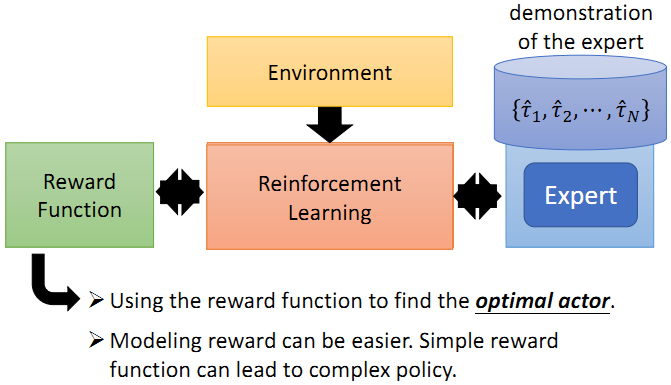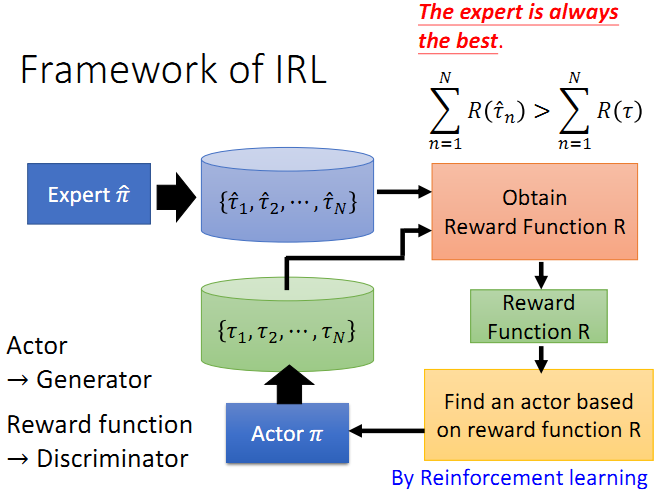1. 专家和actor都会生成对应的 trajectory.
2. 生成的reward function需要满足专家的累积reward总是比actor的大
3. 使用reward function来训练一个新的actor替换原来旧的actor
4. 重复上述步骤.

(假设：专家永远是最棒的)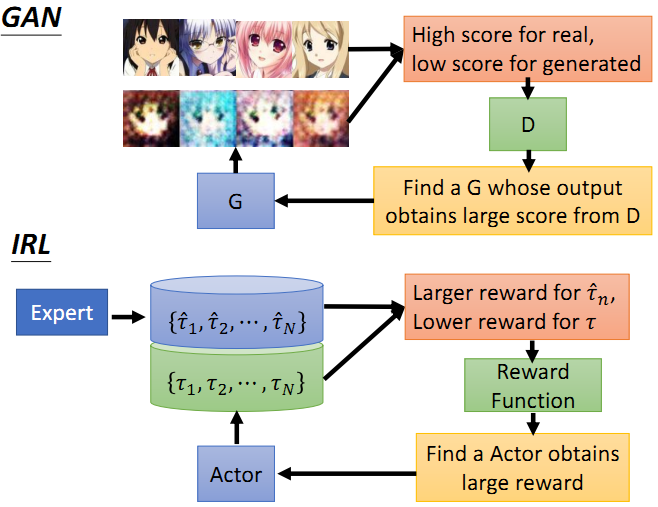2019-02-27 21:07:29 cindy_1102 阅读数 1059

# Reward Shaping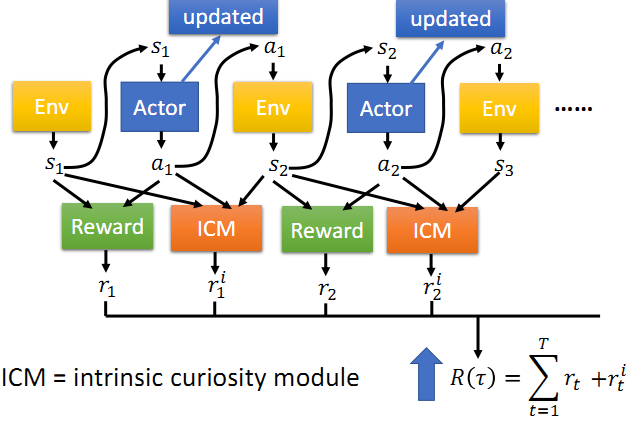ICM函数$s_t, a_t, s_{t+1}$ 作为输入，得到一种新的reward $r^i$，actor的目标现在变为最大化两个部分的reward，即 $r$$r^i$。可以理解为，新的模型中，actor被鼓励基于好奇去采取不一样的行动，这样的好奇带来的行动可以给actor带来额外的收益。

ICM函数的全称为 Intrinsic curiosity module，是用来为actor生成好奇感的函数，它的网络结构如下：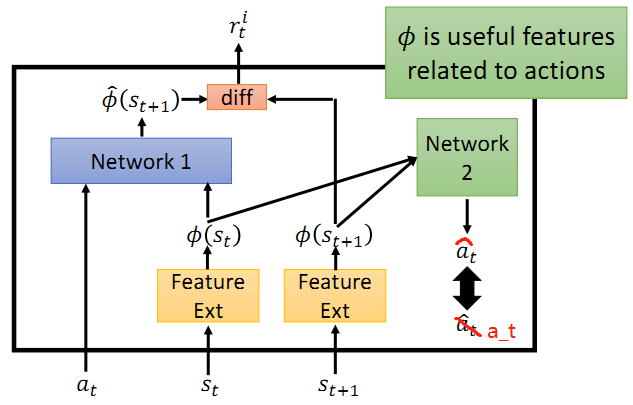Network 1：将提取的$a_t$$s_t$ 的特征作为输入，输出 $s_{t+1}$ 的提取特征的估计。然后真实的 $s_{t+1}$ 的提取特征用来与估计值比较，得到两者之间的差别。两者差别越大则reward $r^i$ 越大，也就是说，模型鼓励actor去冒险。

Network2:：用来提取与action相关的游泳的特征，输入 $s_t$$s_{t+1}$$\phi$ 值，输出估计的action $\hat{a_t}$，如果估计的action和真实的action $a_t$ 接近，则 $\phi$ 可以用来提取有用的特征。

# 课程式学习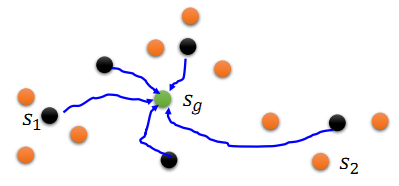# 级联强化学习

2019-02-27 20:13:15 cindy_1102 阅读数 1909

### 李宏毅深度强化学习- Actor-Critic

1. 给定某个state采取某个action的概率
2. 考虑到行动输出的概率和为一，为了保证reward越大的有更大的概率被sample到，需要加上baseline b
3. 考虑到当先action对过去的reward没有影响，从当前时间点开始进行reward的累加
4. 考虑到时间越久，当前action对后期reward的影响越小，添加折扣系数$\gamma$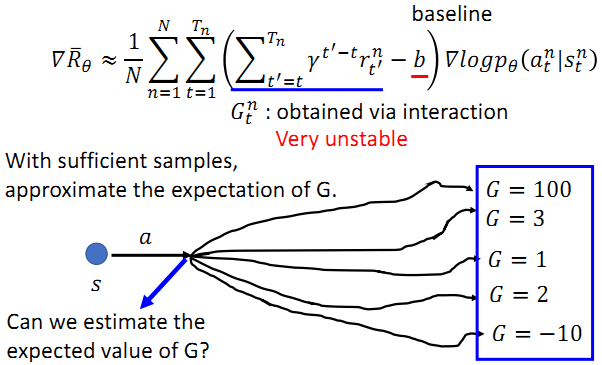## 回顾 – Q-Learning

Q-learning部分主要记住以下几个点：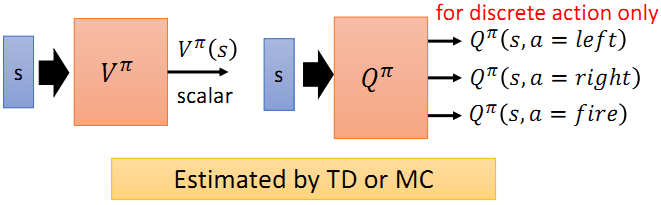## Actor-Critic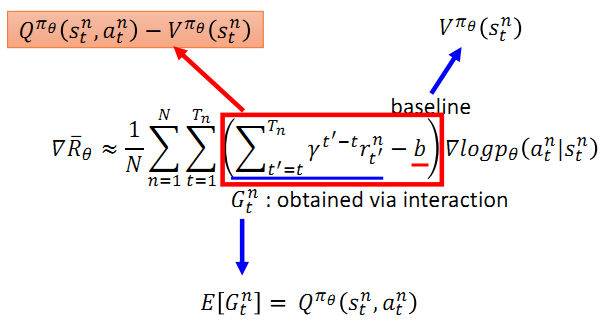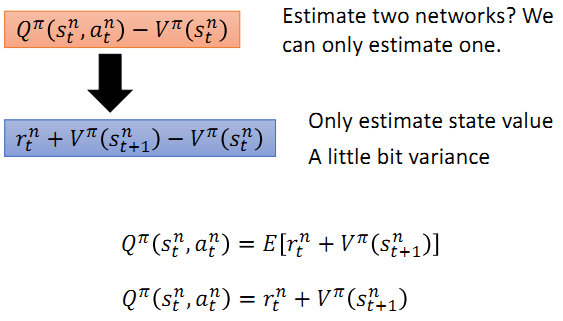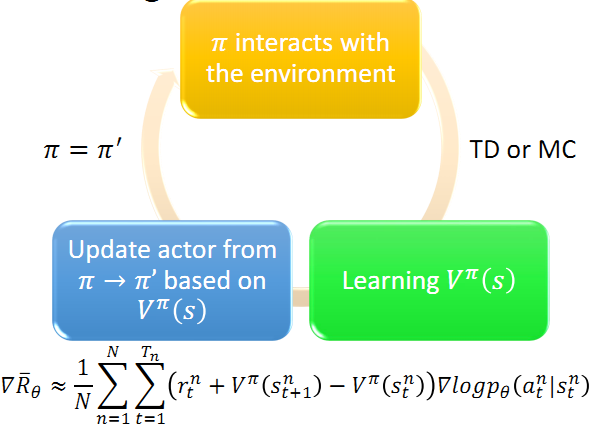actor $\pi(s)$'s 和 critic $V^\pi(s)$ 网络的前几层的参数是可以共享的，因为他们具有相同的输入s，在对s的处理上可以共享该部分参数。

1. 每个worker都会copy全局参数
2. 每个worker都与环境进行互动，并得到sample data
3. 计算梯度
4. 更新全局参数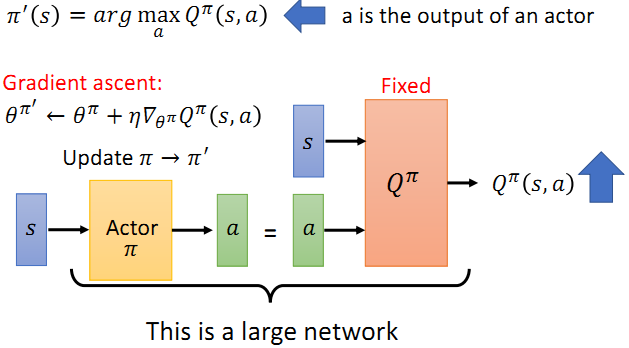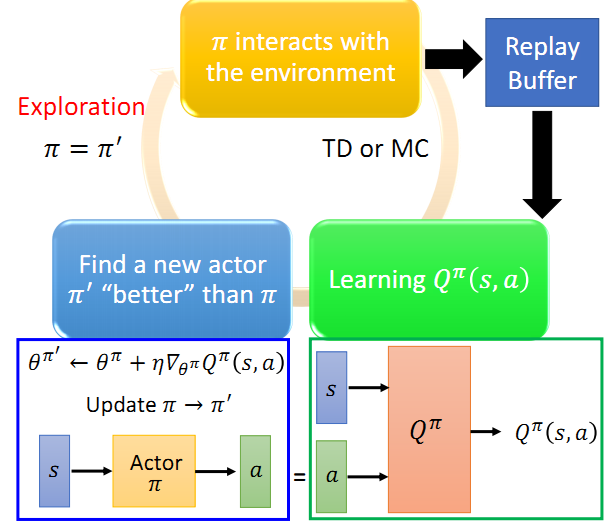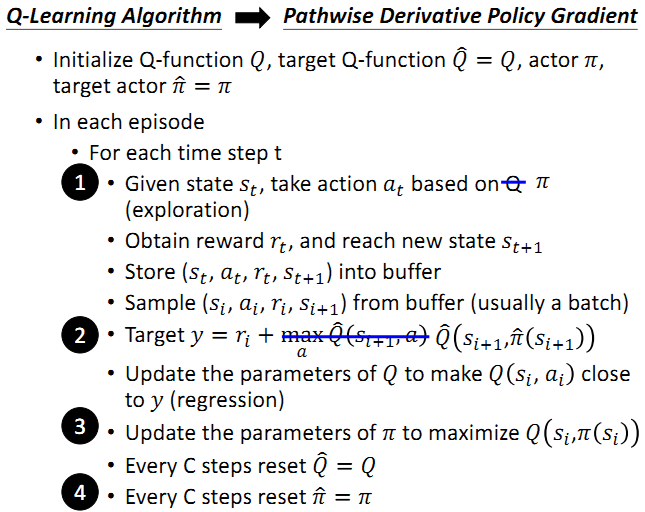1. 当前采取的action由训练的actor决定
2. Q是用state s以及 $\hat{\pi}$ 采取的action来计算的
3. 不仅需要更新Q，也需要更新 $\pi$.